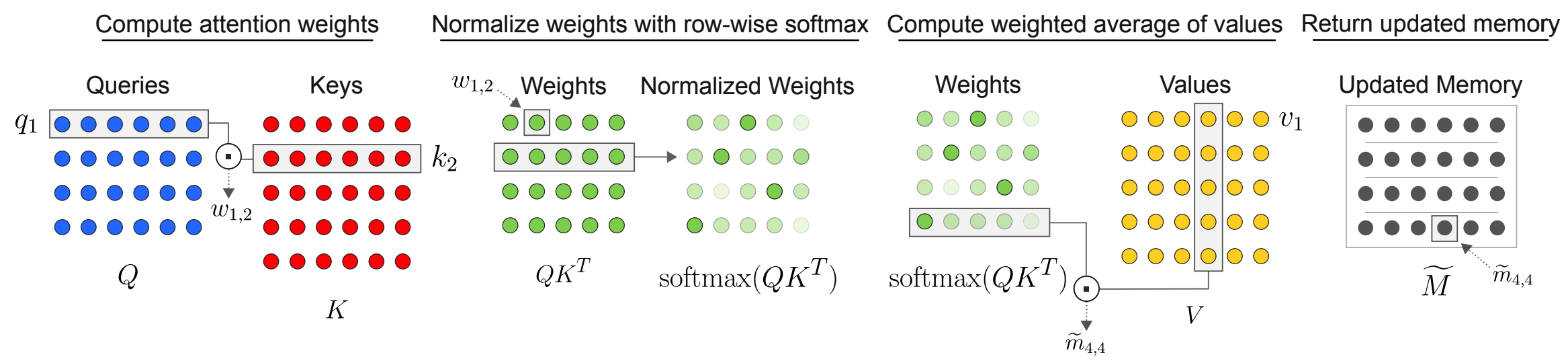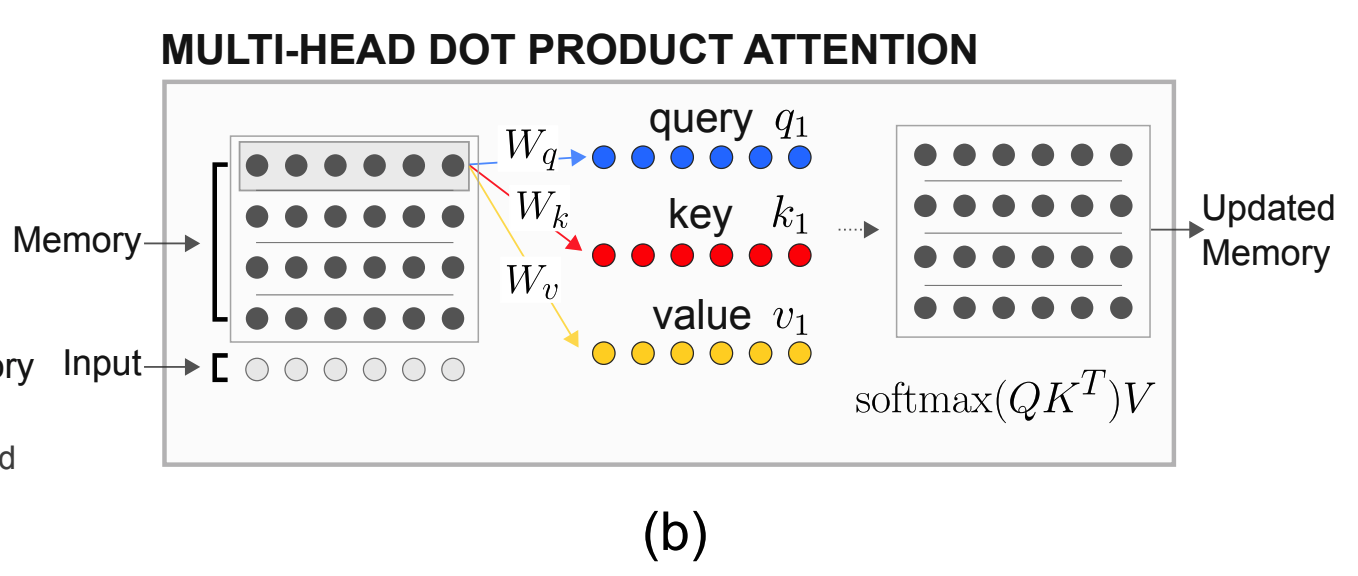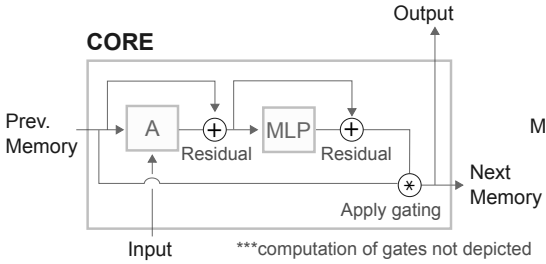# Relational Recurrent Neural Network

## WHY?

While conventional deep learning models have performed well on inference regarding individual entities, few models have been proposed focusing on inference regarding the relations among entities. Relational network aimed to capture relational information from images. In this paper, relational recurrent neural network tried to improve former memory augmented neural network models to capture relations among memories.

## WHAT?

Assume that we have memory matrix M. To capture interaction among these memories, Multi-Head Dot Product Attention(MHDPA) is used (also known as self-attention).Linear projections are used to make queries(Q), keys(K) and values(V) for each memory. Dot product of queries and keys are normalized to gain attention weights. Weighted average of values are used as next memory.

Q = MW^q\\
K = MW^k\\
V = MW^v\\
A_{\theta}(M) = softmax(\frac{MW^q(MW^k)^T}{\sqrt{d_k}})MW^v = \tilde{M}

This composes one head of MHDPA. Assuming h is the number of head in MHDPA, F dimensional memories are projected to F/h dimension for each head, and heads are concatnated to represent next memory. New inputs for memory can be encoded easily without adding more parameters.\tilde{M} = softmax(\frac{MW^q([M;x]W^k)^T}{\sqrt{d^k}})[M;x]W^v

Resulted memory can be used as memory cell in LSTM. row-wise MLP with layer normalization is used for g_{\psi}$g_{\psi}$.s_{i,t} = (h_{i,t-1}, m_{i,t-1})\\
f_{i,t} = W^f x_t + U^f h_{i,t-1} + b^f\\
i_{i,t} = W^i x_t + U^i h_{i,t-1} + b^i\\
o_{i,t} = W^o x_t + U^o h_{i,t-1} + b^o\\
m_{i,t} = \sigma(f_{i,t} + \tilde{b}^f)\circ m_{i, t-1} + \sigma(i_{i,t})\circ g_{\psi}(\tilde{m}_{i,t})\\
h_{i,t} = \sigma(o_{i,t})\circ tanh(m_{i,t})\\
s_{i,t+1} = (m_{i,t}, h_{i,t})

## So?

RRN achieved good performance in Nth farthest vector calculation task, program evaluation, reinforcement learning task and language modeling.

## Critic

Beautiful, but not sure how this work. Need more experiments.

Powered by aiden